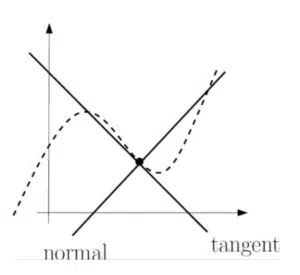# Tangents and Normals at a given pointTangents and normals
are lines at a given point on a curve. A tangent runs parallel with the curve at the point whereas the normal is perpendicular to the curve. It follows that if the curve has gradient m, the tangent also has gradient m and the normal has gradient $-1/m$. See more on perpendicular straight lines.

Tangents and normals, like any other straight lines, have equations of the form $y=mx+c$. Their equations can be found just like any other straight lines. However, you are likely to need the derivative by differentiating when finding the gradient of tangents and normals to a curve.

## Tangents

As mentioned above, the TANGENT to a curve at a given point has the same gradient as the curve at that point. In order to find the gradient of the curve, we require the derivative. This is found by differentiating and obtaining an expression for $dy/dx$. Substituting in an $x$-value will give the gradient of the curve and hence the gradient of the tangent. To find the full equation of the tangent, it remains to find the $y$-intercept of the tangent. This can be achieved using the coordinates of the point that the tangent and the curve have in common.

See Examples 1 and 2. Page 13 onwards of the StudyWell Differentiation eGuide has more on Tangents & Normals including some exam-style questions.

## Normals

The NORMAL to a point on a curve is the line that is perpendicular to the tangent at the given point. If the tangent has a gradient of $m$, the normal has a gradient of $-\frac{1}{m}$. The $y$-intercept of the normal, which is different from that of the tangent, can also be found using the coordinates of the given point.

See Examples 3 and 4. Page 13 onwards of the StudyWell Differentiation eGuide has more on Tangents & Normals including some exam-style questions.

## Examples of Tangents & Normals

Find the equation of the tangent to

$y=x^2$

at the point $(3,9)$.

The curve $C$ has equation $y=2x^3+x^2-3x-7$. The point $P$ with $x$-coordinate -0.5 lies on $C$ and the tangent to $C$ at $P$ is parallel to the straight line $2y-3x+4=0$. Find the equation of the tangent at $P$ in the form $ax+by+c=0$ where $a$, $b$ and $c$ are integers.

Find the equation of the normal to

$y=x^2$

at the point $(3,9)$.

The points $A$ and $B$ lie on the curve with equation $y=f(x)$. Given that $f'(x)=\frac{{x}}{3}-\frac{2}{{x}}+\frac{7}{3}$ and the normals at $A$ and $B$ are both parallel to the line $2y+x=5$, find the $x$-coordinates of $A$ and $B$.